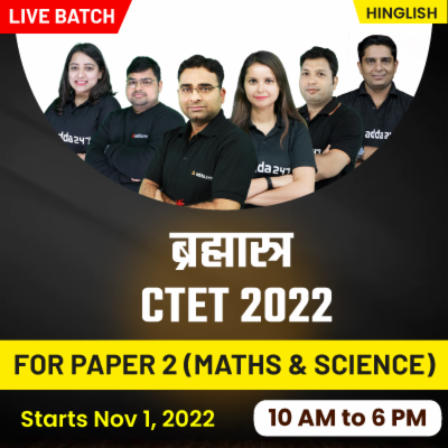Latest Teaching jobs   »   CTET Notification 2023   »   Mathematics Study Notes

# Mathematics Study Notes For All Teaching Exams

Mathematics Study Notes is an equally important section for CTET, HTET,NVS, KVS & DSSSB Exams and has even more abundant importance in some other exams conducted by central or state govt. Mathematics Study Notes will carries various weightage depends on exam. Candidate who are preparing for teaching exams, always fear for Math section.

Here we are providing Mathematics Study Notes of various topics i.e. Discount, Profit loss, Simple Interest, Average, Mensuration, Geometry, Pedagogy etc. Candidate can prepare their Mathematics section prepare with these notes which will make their preparation easy and scoring.

CTET Study Plan

## Mathematics Study Notes Highlights

Mathematics is the crucial subject for clearing the TET and CTET exam which comes in two forms Paper-1 (For Primary Level) and Paper-2 (Secondary Level).

• The mathematics section in TET paper 1 and paper 2 exam has 30 questions for 30 marks in each paper.
• In Paper I, 15 Questions covers content part which is related to Class 1-5 math books and other 15 Questions covers Teaching pedagogy part which is related to teaching methodologies, remedial teaching, errors and evaluation based on Class 1-5 students.
• In Paper II, 15 Questions covers content part which is related to Class 6-8 math books and other 15 Questions covers Teaching pedagogy part which is related to teaching methodologies, remedial teaching, errors and evaluation based on Class 6-8 students.

## Mathematics Section Weightage in Teaching Exams

 Exam Total Marks Total Question CTET PAPER 1 30 30 CTET PAPER 2 30 30 HTET Paper 1 30 30 HTET Paper 2 30 30 DSSSB PRT 20 20 DSSSB TGT Maths 120 120

To let you make the most of Mathematics section, we are providing important facts related to the Important Mathematics Topics. We wish you all the best of luck to come over the fear of the Mathematics section.

Mathematics Study Notes PDF has been given below in PDF format. The details of Mathematics Study Notes has been explained in details.

 S.NO TOPIC 1. Number System 2. Facts and Formulae 3. Properties of Integers 4. Divisibility Rule 5. Powers, Indices and Surds 6. Fractions 7. Linear Equation 8. Algebra 9. Percentage 10. Average 11. Mixture and Allegation 12. Ratio and Proportion 13. Simple Interest 14. Compound Interest 15. Time and Work 16. Speed Distance and Time 17. Lines and Angles 18. Shapes and Spatial Understanding 19. Congruence And Similarity Of Triangles 20. Properties Of Circle 21. Properties Of Circle Part 2 22. Mensuration-2D 23. Mensuration-3D 24. Data Handling 25. Unit Digit 26. Simplification Tricks 27. Basics Of Probability 28. Fundamental Concepts Of Geometry

## Mathematics Previous Year Questions

 S.NO TOPIC 1. CTET 2012 Maths Pedagogy Questions 2. CTET 2014 Maths Pedagogy Questions 3. CTET 2015 Maths Pedagogy Questions 4. CTET 2016 Maths Pedagogy Questions

## Mathematics Practice Quiz

 S.No Click on the link given below to download Maths question PDF for Teaching Exams: 1 Mathematics Miscellaneous Quiz 2. Mathematics Miscellaneous Quiz 3. Mathematics Miscellaneous Quiz 4. Mathematics Miscellaneous Quiz 5. Mathematics Miscellaneous Quiz 6. Maths Mega Quiz In English Maths Mega Quiz In Hindi 7. Maths Mega Quiz In English Maths Mega Quiz In Hindi 8. Maths Mega Quiz In English Maths Mega Quiz In Hindi 9. Maths Mega Quiz In English Maths Mega Quiz In Hindi